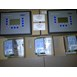Hi, Guest!
• 0

LOKASI :  Jakarta Pusat

AKTIVITAS :  17 hours ago

BAGIKAN :

Bagikan :
SUMBER BERKAT ABADI GOOD QUALITY WITH COMPETITIVE PRICE. GUARANTEE YOU SATISFIED 100%

## Fuse ETI 100 A 24 KV 50 N - 004255015

Update Terakhir
:
19 / 07 / 2020
Min. Pembelian
:
1 Unit
Harga Mulai
Rp. 3.588.000
Sampai dengan
Rp. 3.688.000
Bagikan
:

### Detail Fuse ETI 100 A 24 KV 50 N - 004255015

FUSE ETI 100A 24KV 63kA 50N - 004255015

004255015 VVC 24kV/100A

ETI Code = 004255015
Description = VVC 24kV/100A
Weight = 5.5kg
Level = VVC
Rated current (A) = 100
Type = VVC
Rated AC voltage (V) = 24.000
Indicatorstriker = 50N
Breaking capacity AC (kA) = 63
Indicatorstriker = 50N
Breaking capacity AC (kA) = 63

Nominal current = 100A
Nominal rated current = 100
Type of fuse status indicator = striker 50N
Breaking capacity = 63kA
Max. rated operation voltage Ue AC = 24000

generatorJl. Pintu Air 3 No. 25-C Pasar Baru, Jakarta Pusat
Indonesia 10710
Jakarta Pusat
13 / 08 / 2020
Hubungi Kami

### Detail Fuse ETI 100 A 24 KV 50 N - 004255015

FUSE ETI 100A 24KV 63kA 50N - 004255015

004255015 VVC 24kV/100A

ETI Code = 004255015
Description = VVC 24kV/100A
Weight = 5.5kg
Level = VVC
Rated current (A) = 100
Type = VVC
Rated AC voltage (V) = 24.000
Indicatorstriker = 50N
Breaking capacity AC (kA) = 63
Indicatorstriker = 50N
Breaking capacity AC (kA) = 63

Nominal current = 100A
Nominal rated current = 100
Type of fuse status indicator = striker 50N
Breaking capacity = 63kA
Max. rated operation voltage Ue AC = 24000

generator